Question

# AB 00 01 11 10 CD 00 0 0 4 1 12 1 8 1...

 AB 00 01 11 10 CD 00 0 0 4 1 12 1 8 1 01 1 1 5 1 13 1 9 1 11 3 1 7 0 15 0 11 0 10 2 0 6 0 14 0 10 1

Simplify F(A, B, C, D) using the zeros of the k-map to get F`, then use De Morgan’s formula to get F in product of sums and select the one that matches it from the following;

a- F(A,B,C,D)= [(A+B`)(C+B`)(B+D`)]

b- F(A,B,C,D)= [(A`+B)(C`+B`)(B+D`)]

c- F(A,B,C,D)=[(A`+D`)(C+B`)(B+D`)]

d- F(A,B,C,D)=[(B`+C`)(A`+C`+D`)(A+B+D)]

e- F(A,B,C,D)=[(A`+B)(C+B`)(B+D`)]

f- NONE of the above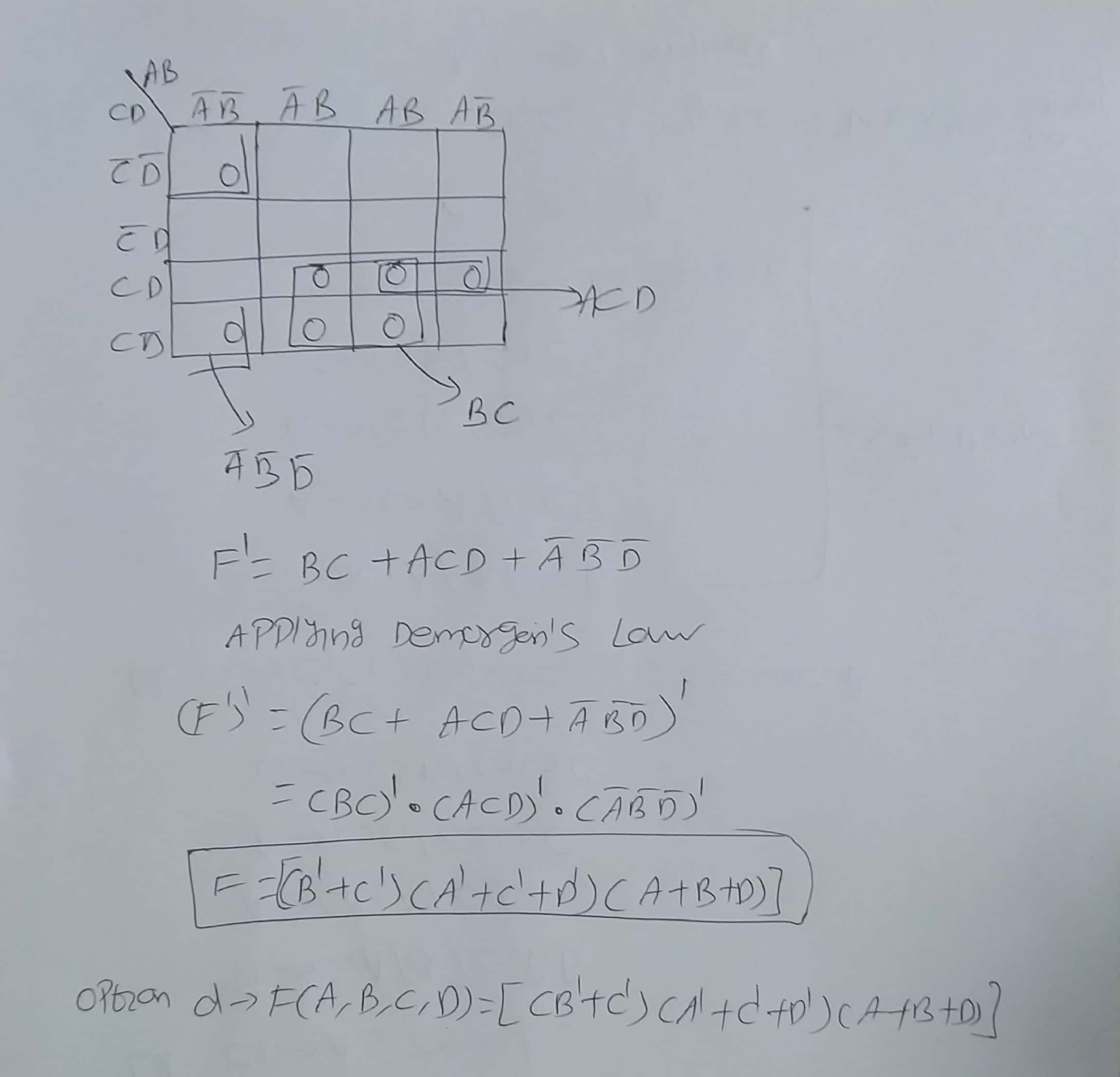thank you and all the best

#### Earn Coins

Coins can be redeemed for fabulous gifts.

Similar Homework Help Questions
• ### Given the following Karnaugh map AB CD 00 01 11 10 01 011 Draw a circuit...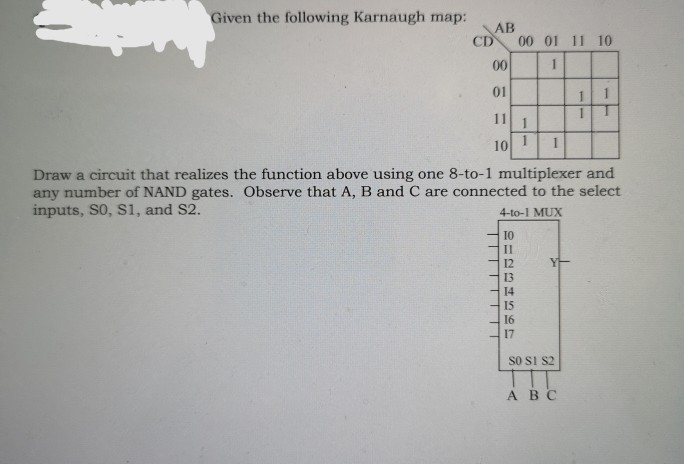Given the following Karnaugh map AB CD 00 01 11 10 01 011 Draw a circuit that realizes the function above using one 8-to-1 multiplexer and any number of NAND gates. Observe that A, B and C are connected to the select inputs, SO, S1, and S2. 4-to-1 MUX -10 12 13 14 15 16 17 So Si S2 ABC

• ### What is the simplified function of the following Karnaugh Map? AB CD 00 01 11 10...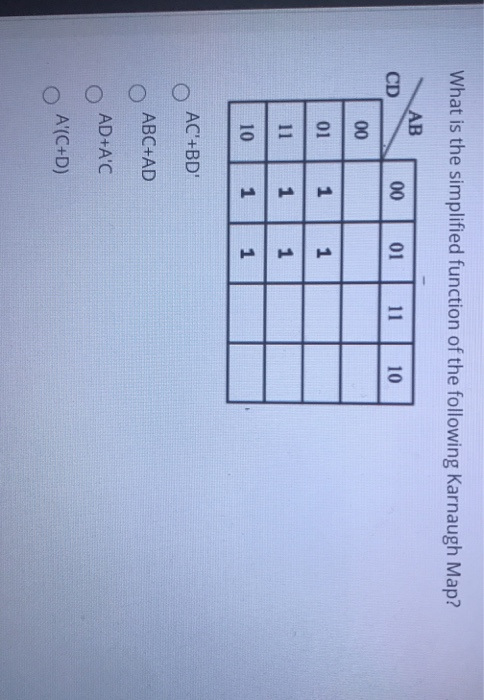What is the simplified function of the following Karnaugh Map? AB CD 00 01 11 10 00 01 1 1 11 1 1 10 1 1 AC'+BD' O ABC+AD AD+A'C A'(C+D)

• ### Simplify the following K-map: F(A,B,C,D,E) = 2(0,1,2,3,8,10,13,15,16,17,18,19,24,26,29) A=0 00 01 11 A=1 DE BC0001 11 10...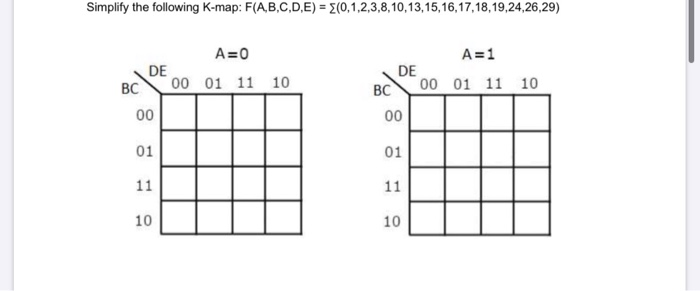Simplify the following K-map: F(A,B,C,D,E) = 2(0,1,2,3,8,10,13,15,16,17,18,19,24,26,29) A=0 00 01 11 A=1 DE BC0001 11 10 10

• ### 1. For the following function: f(a, b, c, d) =>m(0, 1, 4, 8, 10, 15)d(2,5,7, 11,...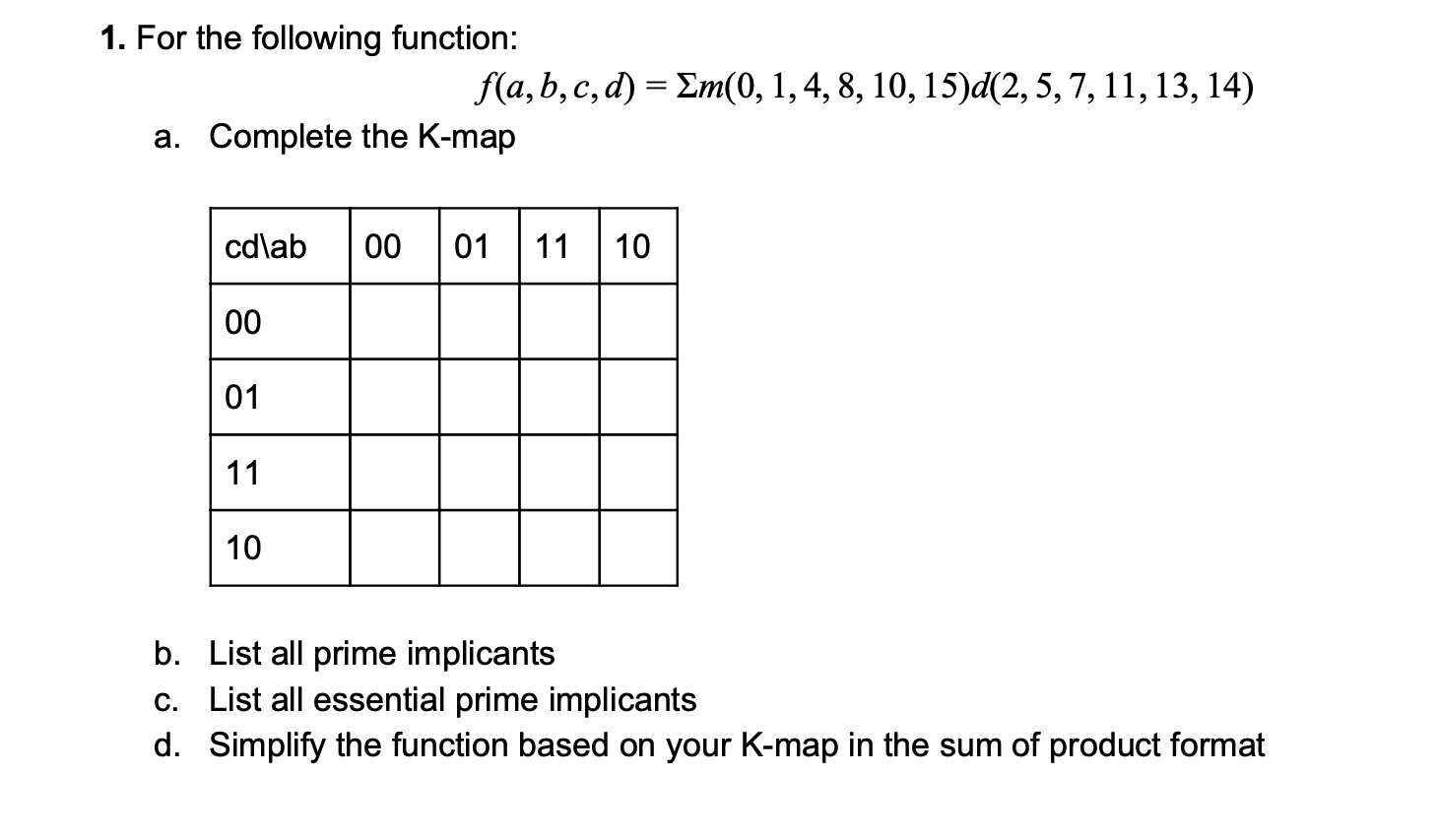1. For the following function: f(a, b, c, d) =>m(0, 1, 4, 8, 10, 15)d(2,5,7, 11, 13, 14) a. Complete the K-map cdlab 00 01 11 10 00 10 b. List all prime implicants c. List all essential prime implicants d. Simplify the function based on your K-map in the sum of product format

• ### What is the minimum sum-of-products expression for the following Kmap? AB 00 01 11 10 CD...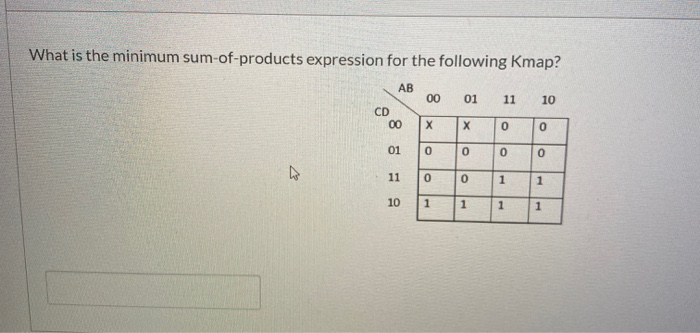What is the minimum sum-of-products expression for the following Kmap? AB 00 01 11 10 CD 00 х х 0 0 01 0 o 0 0 11 0 0 1 1 10 1 1 1 1

• ### Simplest SOP AD 00 01 11 10 cD or O d d loSimplest POS 10 Minimize...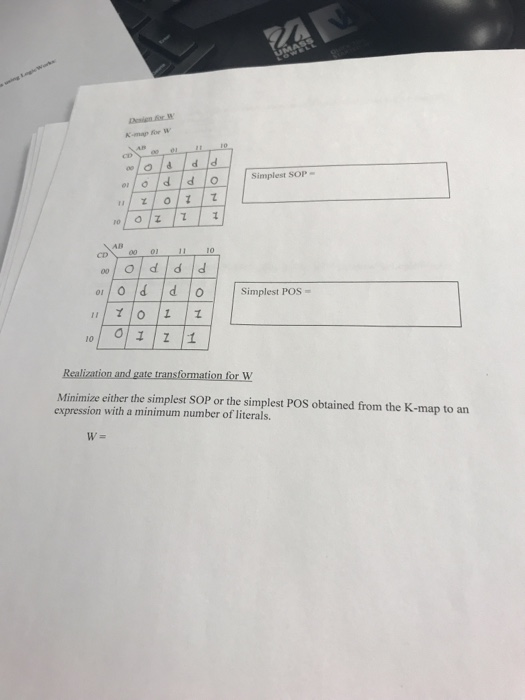Simplest SOP AD 00 01 11 10 cD or O d d loSimplest POS 10 Minimize either the simplest SOP or the simplest POS obtained from the K-map to an expression with a minimum number of literals.

• ### help!!! Given the following expression: F1 (A,B,C,D) -A'BC+ABC+CD+ACD Select the correct K-map: a. AB CD 01...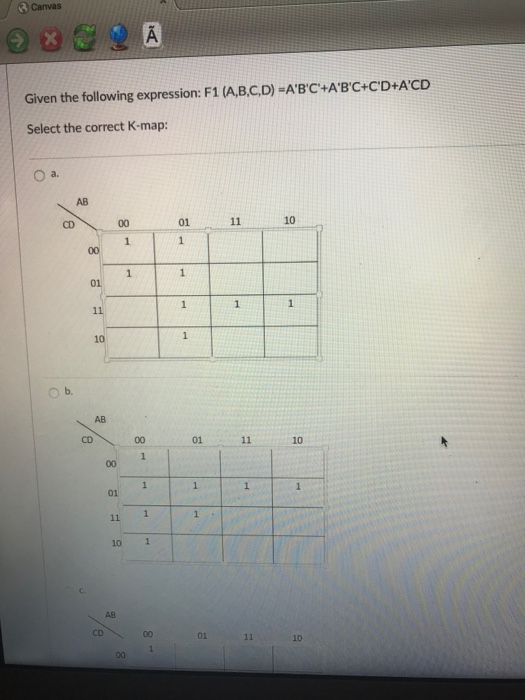help!!! Given the following expression: F1 (A,B,C,D) -A'BC+ABC+CD+ACD Select the correct K-map: a. AB CD 01 10 10 ○b. AB CD 0111 10 10 1 01

• ### DI Question 6 2 pts Consider the following Truth table 000 0| 0 000 11 00 10| 0 00 1 11 0100|1 01 0 11 0 01 1 0 | 0 01 1 1| 0 100 0 | 0 100 1| 1 10 1010 10 1 1| 1 11 001 O 11 0 1 1 0 Fill the followi...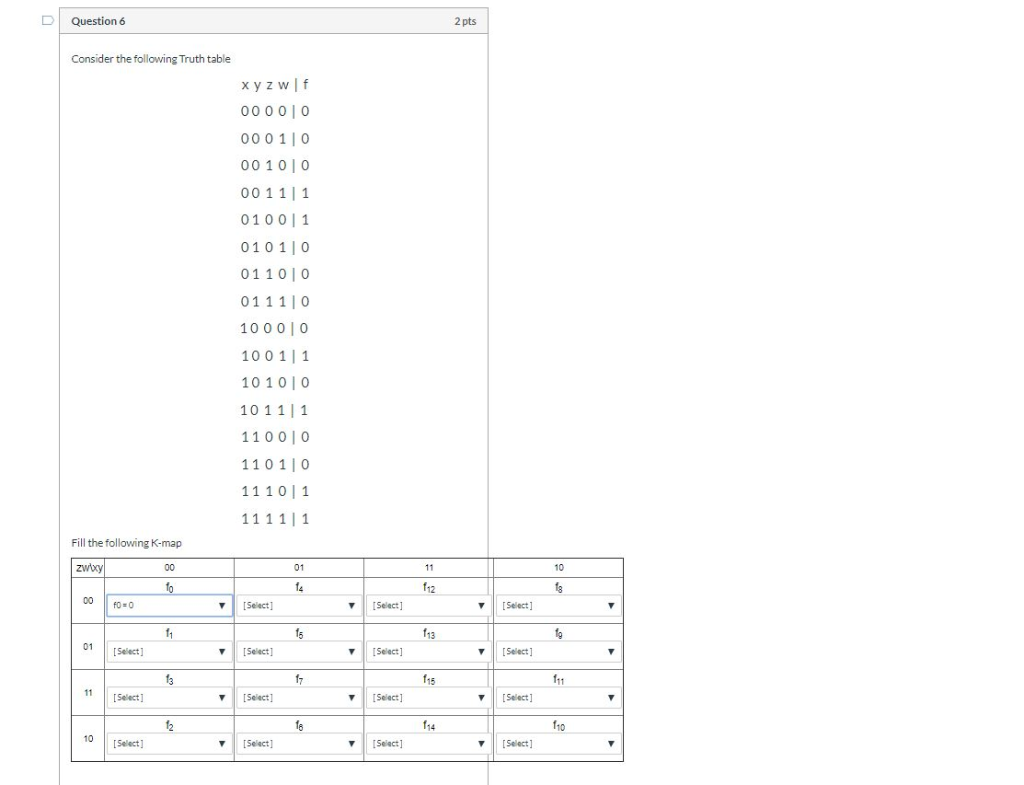DI Question 6 2 pts Consider the following Truth table 000 0| 0 000 11 00 10| 0 00 1 11 0100|1 01 0 11 0 01 1 0 | 0 01 1 1| 0 100 0 | 0 100 1| 1 10 1010 10 1 1| 1 11 001 O 11 0 1 1 0 Fill the following K-map 01 2 Select ▼ | [Select] 01 sect] | ▼ | [Select] ▼ | [Select] [Select] f11 15 | ▼...

• ### Question 9 What is the minimum sum-of-products expression for the following Kmap? AB 00 01 11...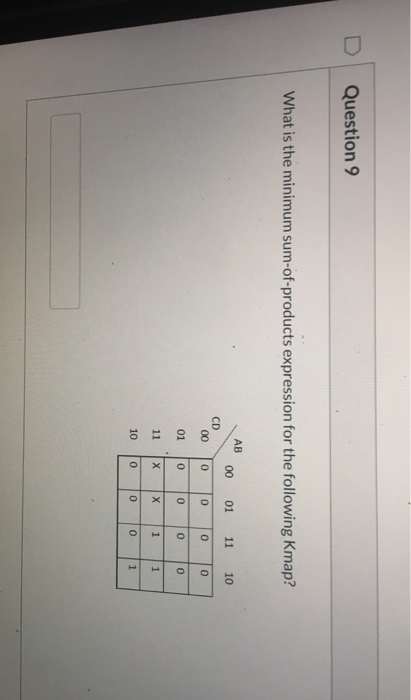Question 9 What is the minimum sum-of-products expression for the following Kmap? AB 00 01 11 10 CD 00 0 0 0 0 01 0 0 0 0 11 X X 1 10 0 0 o 1

• ### Please show the detailed proof clearly.. 1. The Karnuagh map results from this Boolean equation AB...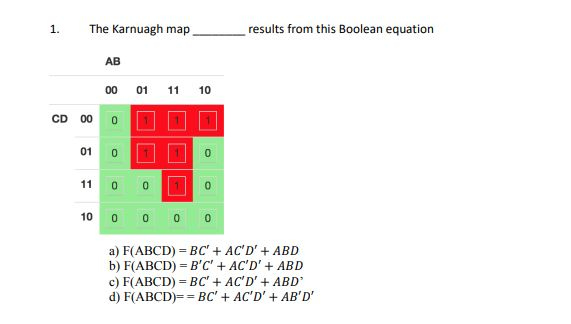Please show the detailed proof clearly.. 1. The Karnuagh map results from this Boolean equation AB 00 01 11 10 CD 8 0 01 0 0 11 0 0 10 0 0 0 0 a) F(ABCD) - BC' + AC'D' + ABD b) F(ABCD) - B'C' + AC'D' + ABD c) F(ABCD) - BC' + AC'D' + ABD' d) F(ABCD)==BC' + AC'D' + AB'D'